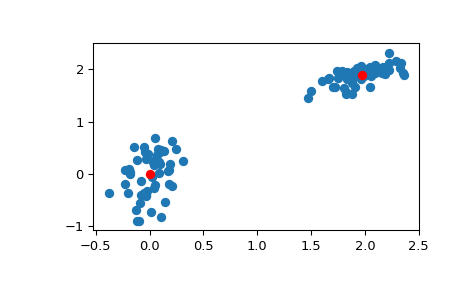# scipy.cluster.vq.kmeans¶

scipy.cluster.vq.kmeans(obs, k_or_guess, iter=20, thresh=1e-05, check_finite=True)[source]

Performs k-means on a set of observation vectors forming k clusters.

The k-means algorithm adjusts the centroids until sufficient progress cannot be made, i.e. the change in distortion since the last iteration is less than some threshold. This yields a code book mapping centroids to codes and vice versa.

Distortion is defined as the sum of the squared differences between the observations and the corresponding centroid.

Parameters: obs : ndarray Each row of the M by N array is an observation vector. The columns are the features seen during each observation. The features must be whitened first with the whiten function. k_or_guess : int or ndarray The number of centroids to generate. A code is assigned to each centroid, which is also the row index of the centroid in the code_book matrix generated. The initial k centroids are chosen by randomly selecting observations from the observation matrix. Alternatively, passing a k by N array specifies the initial k centroids. iter : int, optional The number of times to run k-means, returning the codebook with the lowest distortion. This argument is ignored if initial centroids are specified with an array for the k_or_guess parameter. This parameter does not represent the number of iterations of the k-means algorithm. thresh : float, optional Terminates the k-means algorithm if the change in distortion since the last k-means iteration is less than or equal to thresh. check_finite : bool, optional Whether to check that the input matrices contain only finite numbers. Disabling may give a performance gain, but may result in problems (crashes, non-termination) if the inputs do contain infinities or NaNs. Default: True codebook : ndarray A k by N array of k centroids. The i’th centroid codebook[i] is represented with the code i. The centroids and codes generated represent the lowest distortion seen, not necessarily the globally minimal distortion. distortion : float The distortion between the observations passed and the centroids generated.

kmeans2
a different implementation of k-means clustering with more methods for generating initial centroids but without using a distortion change threshold as a stopping criterion.
whiten
must be called prior to passing an observation matrix to kmeans.

Examples

>>> from numpy import array
>>> from scipy.cluster.vq import vq, kmeans, whiten
>>> import matplotlib.pyplot as plt
>>> features  = array([[ 1.9,2.3],
...                    [ 1.5,2.5],
...                    [ 0.8,0.6],
...                    [ 0.4,1.8],
...                    [ 0.1,0.1],
...                    [ 0.2,1.8],
...                    [ 2.0,0.5],
...                    [ 0.3,1.5],
...                    [ 1.0,1.0]])
>>> whitened = whiten(features)
>>> book = np.array((whitened,whitened))
>>> kmeans(whitened,book)
(array([[ 2.3110306 ,  2.86287398],    # random
[ 0.93218041,  1.24398691]]), 0.85684700941625547)

>>> from numpy import random
>>> random.seed((1000,2000))
>>> codes = 3
>>> kmeans(whitened,codes)
(array([[ 2.3110306 ,  2.86287398],    # random
[ 1.32544402,  0.65607529],
[ 0.40782893,  2.02786907]]), 0.5196582527686241)

>>> # Create 50 datapoints in two clusters a and b
>>> pts = 50
>>> a = np.random.multivariate_normal([0, 0], [[4, 1], [1, 4]], size=pts)
>>> b = np.random.multivariate_normal([30, 10],
...                                   [[10, 2], [2, 1]],
...                                   size=pts)
>>> features = np.concatenate((a, b))
>>> # Whiten data
>>> whitened = whiten(features)
>>> # Find 2 clusters in the data
>>> codebook, distortion = kmeans(whitened, 2)
>>> # Plot whitened data and cluster centers in red
>>> plt.scatter(whitened[:, 0], whitened[:, 1])
>>> plt.scatter(codebook[:, 0], codebook[:, 1], c='r')
>>> plt.show()#### Previous topic

scipy.cluster.vq.vq

#### Next topic

scipy.cluster.vq.kmeans2